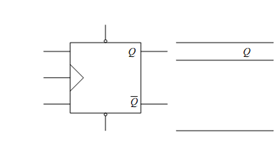## Preset and Clear Inputs Assignment Help

Assignment Help: >> Flip-Flops - Preset and Clear Inputs

Preset and Clear Inputs:

Usually JK Flip Flops are clocked Flip Flops. But we may add PRESET and CLEAR inputs to the JK Flip Flops as illustrated in Figure (a). They are negative enabled inputs that mean they shall be active only when they are low. These inputs override the other states of the Flip Flops and they are called as asynchronous or forced inputs. If PR = 0 and CL = 1, then Q shall become 1 no matter what are the values of J and K. Likewise, if PR = 1 and CL = 0, then Q shall change to 0 for any values of J and K inputs. Only whereas PR = 1 and CL = 1, these forced or asynchronous inputs are disabled and it shall behave like the normal JK Flip Flop. But the case when PR = 0 and CL = 0 is forbidden since that shall make Q = 1 and Q¯ = 1. All of these states of JK Flip Flops with PR and CL inputs are summarized in the truth table of Figure (b).(a)                            (b)

Figure: (a) JK Type Flip Flop with PR and CLR Asynchronous Inputs, and (b) Truth Table